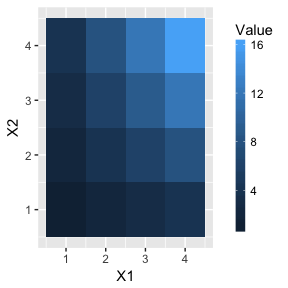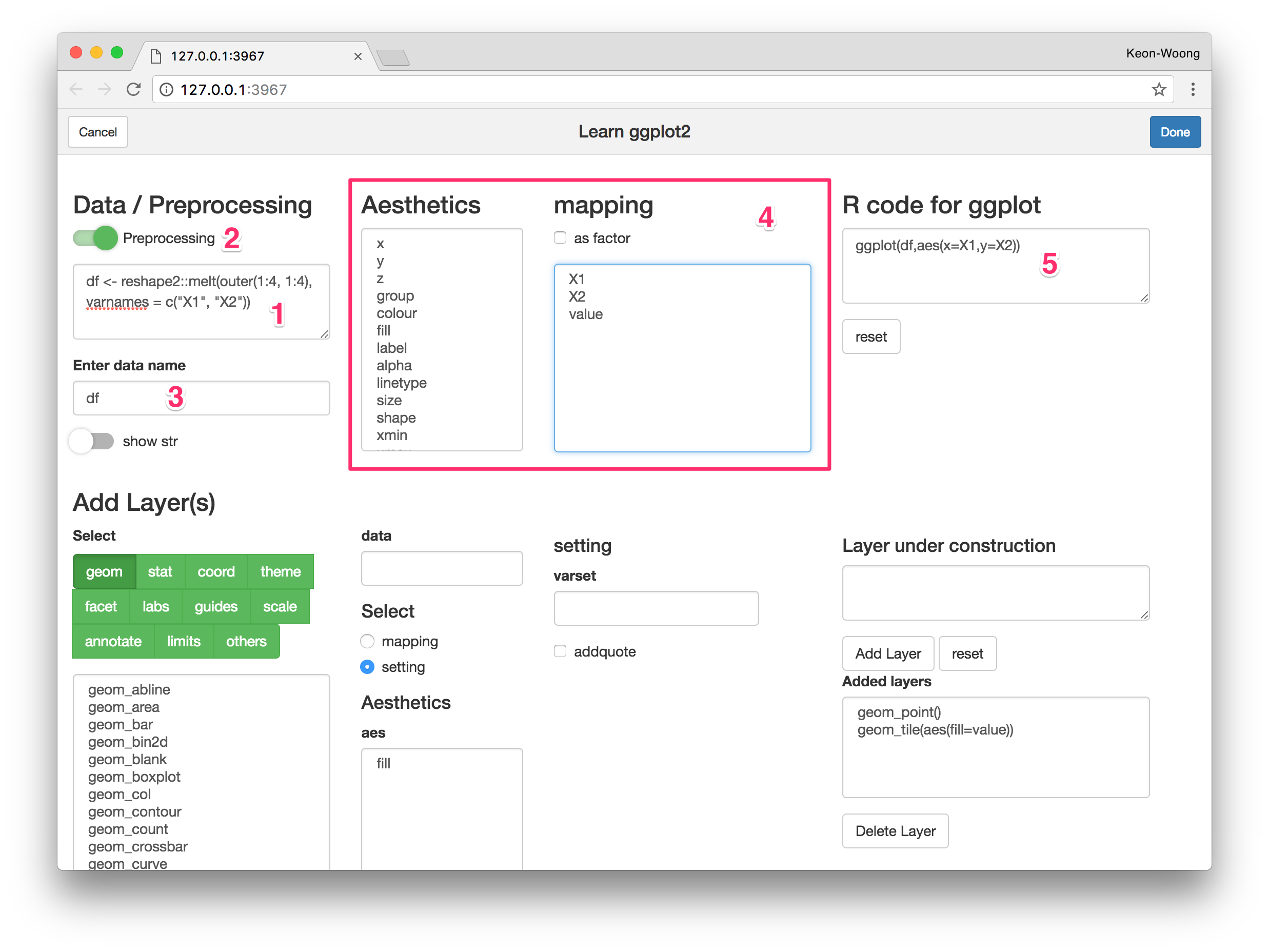How to use guide_colourbar()?

R package ggplotAssist Example(1)

2017-10-25

In this vignette, I’d like to explain how to use guide_colourbar() function to control color bar.

Prerequisite

If you are not familiar with the R package ggplotAssist, please read the following REAME file of the ggplotAssist package.

Install package

You can install developmental version of ggplotAssist package from github.

#install.packages("devtools")
devtools::install_github("cardiomoon/ggplotAssist")

guide_colourbar() function

You can see help file for guide_colourvar() function by typing ?guide_colourbar in R console. This function has so many arguments.

guide_colourbar(title = waiver(), title.position = NULL,
title.theme = NULL, title.hjust = NULL, title.vjust = NULL,
label = TRUE, label.position = NULL, label.theme = NULL,
label.hjust = NULL, label.vjust = NULL, barwidth = NULL,
barheight = NULL, nbin = 20, raster = TRUE, ticks = TRUE,
draw.ulim = TRUE, draw.llim = TRUE, direction = NULL,
default.unit = "line", reverse = FALSE, order = 0, ...)

Example in R help file

We are going to make this plot.

require(ggplot2)
df <- reshape2::melt(outer(1:4, 1:4), varnames = c("X1", "X2"))
ggplot(df, aes(X1, X2)) +
geom_tile(aes(fill = value)) +
guides(fill = guide_colorbar(barwidth = 0.5, barheight = 10, title="Value"))Preprocessing

1. Launch the ggplotAssist Add-in from RStudio. Type the following R code in preprocesing textInput(1).
df <- reshape2::melt(outer(1:4, 1:4), varnames = c("X1", "X2"))

Turn on the preprocessing(2). Enter df as a data name(3). Select x among Aesthetics and select X1 among mapping. And then select y and X2 to make R code for ggplot(5).Make geom_tile() layer

Select geom among layers(1). Select geom_tile function(2). Select mapping radioButton(3). Select fill among aesthetics(4) and assign value(5). Confirm the R code for layer(6) and press Add Layer button(7).